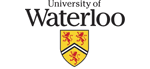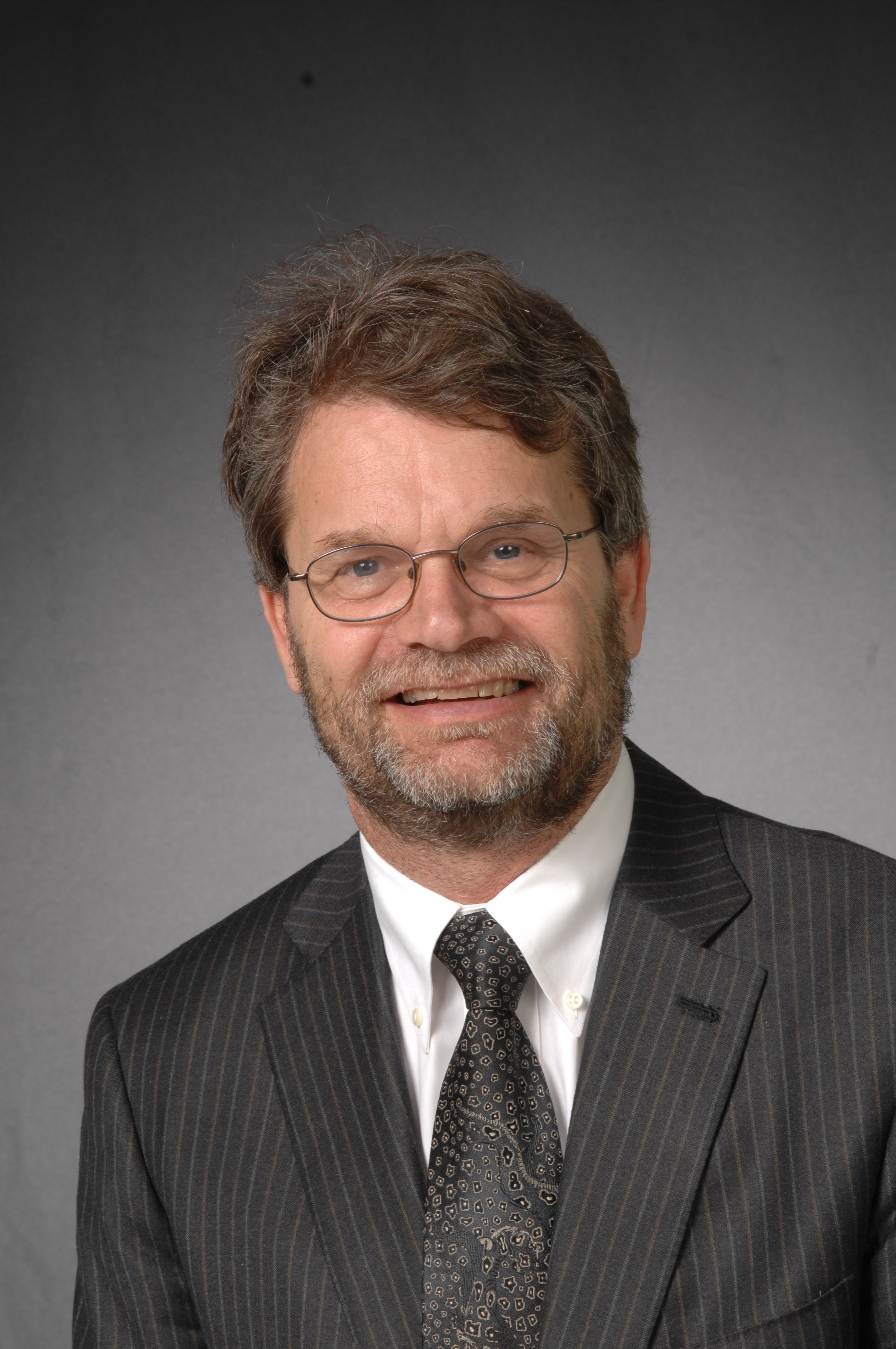# George LabahnDirector Symbolic Computation Group Professor David R. Cheriton School of Computer Science University of Waterloo Ontario, Canada, N2L 3G1 Office: DC3629 Phone: 519-888-4567 x34667 Fax: 519-885-1208 Email: glabahn@uwaterloo.ca

I am interested in many areas of computational mathematics. My main area of research lies in the field of Computer Algebra with a secondary area being in the field of Computational Finance. In addition, I am the principal researcher in the MathBrush project, which seeks to create software for doing mathematics on pen-based devices.

My work on computer algebra centers on effective algorithms for both exact and symbolic-numeric computation. My work includes algorithms for Matrix Normal Forms (polynomial and Ore domains), Greatest Common Divisors of numeric polynomials, Differential equations (particularly those with elliptic functions as coefficients) and Rational Approximation and Interpolation. My main co-author in this topic (and numerous other topics) is Bernhard Beckermann

My work in computational finance centers on fast, stable methods for solving financial option problems, particularly options whose underlying asset follows a jump diffusion pricing model. My main co-author in this topic is Peter Forsyth

My work on pen-based mathematics centers on the development of the MathBrush penmath system. This work includes recognition of handwritten mathematics along with understanding how mathematics can be done using only a pen and a computer algebra backend,.

I am an associate editor of Journal of Symbolic Computation (JSC). I am a former associate editor of ACM Transactions of Mathematical Software (TOMS).

• My CV
• Publications list
• Talks

### Some Specific Research Topics

• Computer Algebra
• Order/Sigma bases [ Ref1, Ref2, Ref3] (with B. Beckermann)
Fast algorithms for Order Bases [ Ref1 Ref2](with W. Zhou)

• Fast algorithms for polynomial matrix arithmetic [ Ref1, Ref2, Ref3, Ref4] (with W. Zhou)

• Fraction-free algorithms for computing matrix GCD's and matrix rational interpolants [ Ref1, Ref2, Ref3] (with B. Beckermann)
Fraction-free algorithms for computing normal forms of polynomial matrices (with B. Beckermann and G. Villard [Ref1, Ref2])
and Ore polynomials (with B. Beckermann and H. Cheng [Ref3]).

• Scaling and finite abelian group symmetries [Ref1,Ref2 , Ref3 ] (with E. Hubert)

• Probabilistic algorithms for Hermite and Smith normal forms of polynomial matrices [Ref1, Ref2, Ref3] (with A. Storjohann)

• Semi-numerical Computations: GCD's of numerical polynomials [Ref1, Ref2] (with B. Beckermann).
The SNAP package (with C-P Jeannerod) [Ref]
Rational functions (with B. Beckermann and A. Matos) [Ref]

• Semi-numerical sparse interpolation of black box polynomials [Ref1, Ref2] ( with M. Giesbrecht and W-s Lee)
and the numerical conditioning of these and related problems [Ref2] (with B. Beckermann and G. Golub).

• Closed form solutions of linear odes having elliptic functions as coefficients (with R. Burger and M. van Hoeij) [Ref].
Hyperexponential solutions of orthogonal systems of Ore operators (with Ziming Li) [Ref]
Differential equations in Maple (old) [Ref]

• Computational Finance
• Pricing of convertable bonds (with Y. d'Halluin, P.A. Forsyth and K. Vetzal [Ref])

• Penalty method for American options with jump diffusion (with Y. d'Halluin and P.A. Forsyth [Ref])

• Asian options with american conditions under jump diffusion processes (with Y. d'Halluin and P.A. Forsyth [Ref])

• Controlled HJB PDEs in Finance (with P.A. Forsyth [Ref])

• Guaranteed Benefit contracts (with A. Belanger and P.A. Forsyth [Ref])

• Singular Control Problems in Finance (with P.A. Forsyth and I. Huang [Ref1, Ref2, Ref3, Ref4])

• Rational Approximation
• Uniform computation of matrix rational approximants (with B. Beckermann) [Ref1, Ref2, Ref3, Ref4, Ref5]

• Fast, numerically stable computation of matrix Pade approximants (with S. Cabay and T. Jones) [Ref1, Ref2, Ref3]
• Structured Matrices
Inversion of structured matrices (with B. Beckermann, D.K. Choi, S. Cabay, T. Shalom) [Ref1, Ref2, Ref3, Ref4, Ref5]

• Pen Math Interfaces
• The MathBrush pen math system [ Ref1, Ref2, Ref3 ]

• Recognizers for handwritten math [ Ref1 , Ref2 ] (with S. Maclean)

• Matrix algebra and pen math input [ Ref ]

• PenMath corpora [Ref]

### Maple Software

• Integration of Elliptic Functions (2004)

• Solving linear ODEs with doubly periodic coefficients (2003,2004)

• The Matrix Polynomial Algebra package in Maple (2002)

• The SNAP package in Maple (with C.P. Jennerod) (2001)

• Special function solutions of higher order linear odes using the Meijer G function (2000)

• Solving linear differential equation using differential factorization (1999-2000)

• Solution of linear differential equations via special functions in Maple (1996-2000)

• The DEtools package for working with differential equations in Maple (1992-1998)

• Computation of indefinite Elliptic integrals in Maple (1996)

• The Plots graphics package in Maple (1990-1995)

• The Plottools graphics package in Maple (1995)

• The inttrans package for computation of integral transforms in Maple (1992-1995)

• Computation of definite Elliptic Integrals in Maple (1991-1996)

• The linear ODE solver in Maple (1993-1998)

• The Mpade package for matrix rational approximation (in Maple share library) (1993)

• Matrix similarity in Maple (1994)

• Frobenius and Jordan Normal Forms in Maple (1992-1995)

• Indefinite Integration of Bessel Functions in Maple (1993)

• Extensions of Risch Algorithm for functions defined by integrals in Maple (1992)

• Implementation of bivariate modular gcd algorithm in Maple (1991)

• Computation of the Matrix Exponential in Maple (1989)

• ## Other Pictures• Miscellaneous: York Tournament